• 如果软件制造商能够可靠地说明对受版权保护的作品的使用是否受到合理使用的保护，那么将会有一条人迹罕至的道路。 但是将机器学习应用于合理使用面临着相当大的障碍。 合理使用已经产生了数百个报告案例，但机器学习...
• 这个有趣的项目展示了如何使用以太坊网络来提供合理的配给卡系统。 您可以在Wikipedia上找到有关配给卡的更多信息： 在Ropsten网络上运行的演示： 设置 安装软件包。 yarn install // or npm install 运行开发区...
• corr学习somquadrotor 用于环境解释的分布式认知图的简单实现。 输入活动会轻微影响... 该网络使用SOM将输入数据编码为人口代码，然后使用Hebbian学习来提取对功能进行编码的共激活模式。 来自四旋翼系统的数据集。MATLAB
• 比DElphi自带的网络控件使用更方便的网络控件，能在多个Delph版本中使用，对UDP有通信支持更科学合理
• ## 神经网络学习 之 BP神经网络

万次阅读 多人点赞 2015-11-30 21:17:56
由这些人工神经元构建出来的网络，才能够具有学习、联想、记忆和模式识别的能力。BP网络就是一种简单的人工神经网络。 本文具体来介绍一下一种非常常见的神经网络模型——反向传播(Back Propagation)神经网络。概述...
上一次我们讲了M-P模型，它实际上就是对单个神经元的一种建模，还不足以模拟人脑神经系统的功能。由这些人工神经元构建出来的网络，才能够具有学习、联想、记忆和模式识别的能力。BP网络就是一种简单的人工神经网络。 本文具体来介绍一下一种非常常见的神经网络模型——反向传播(Back Propagation)神经网络。
概述
BP（Back Propagation）神经网络是1986年由Rumelhart和McCelland为首的科研小组提出，参见他们发表在Nature上的论文 Learning representations by back-propagating errors 。
BP神经网络是一种按误差逆传播算法训练的多层前馈网络，是目前应用最广泛的神经网络模型之一。BP网络能学习和存贮大量的 输入-输出模式映射关系，而无需事前揭示描述这种映射关系的数学方程。它的学习规则是使用最速下降法，通过反向传播来不断 调整网络的权值和阈值，使网络的误差平方和最小。
BP算法的基本思想
上一次我们说到，多层感知器在如何获取隐层的权值的问题上遇到了瓶颈。既然我们无法直接得到隐层的权值，能否先通过输出层得到输出结果和期望输出的误差来间接调整隐层的权值呢？BP算法就是采用这样的思想设计出来的算法，它的基本思想是,学习过程由信号的正向传播与误差的反向传播两个过程组成。
正向传播时，输入样本从输入层传入,经各隐层逐层处理后,传向输出层。若输出层的实际输出与期望的输出(教师信号)不符,则转入误差的反向传播阶段。反向传播时，将输出以某种形式通过隐层向输入层逐层反传,并将误差分摊给各层的所有单元,从而获得各层单元的误差信号,此误差信号即作为修正各单元权值的依据。
这两个过程的具体流程会在后文介绍。
BP算法的信号流向图如下图所示BP网络特性分析——BP三要素
我们分析一个ANN时，通常都是从它的三要素入手，即 1)网络拓扑结构； 2)传递函数； 3)学习算法。每一个要素的特性加起来就决定了这个ANN的功能特性。所以，我们也从这三要素入手对BP网络的研究。
3.1 BP网络的拓扑结构
上一次已经说了，BP网络实际上就是多层感知器，因此它的拓扑结构和多层感知器的拓扑结构相同。由于单隐层（三层）感知器已经能够解决简单的非线性问题，因此应用最为普遍。三层感知器的拓扑结构如下图所示。 一个最简单的三层BP：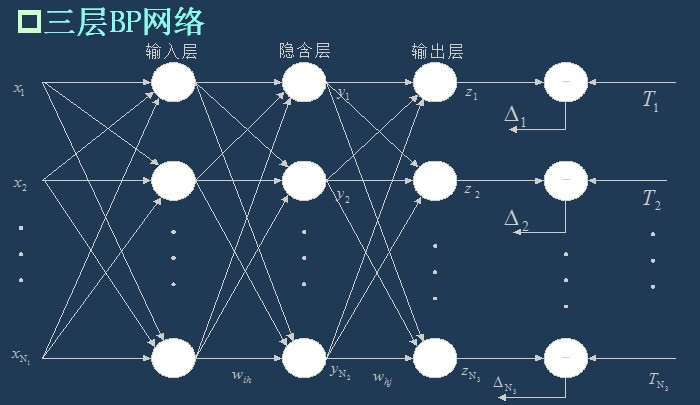###3.2 BP网络的传递函数 BP网络采用的传递函数是非线性变换函数——Sigmoid函数（又称S函数）。其特点是函数本身及其导数都是连续的，因而在处理上十分方便。为什么要选择这个函数，等下在介绍BP网络的学习算法的时候会进行进一步的介绍。 单极性S型函数曲线如下图所示。

f

(

x

)

=

1

1

+

e

−

x

f(x)={1\over 1+e^{-x}}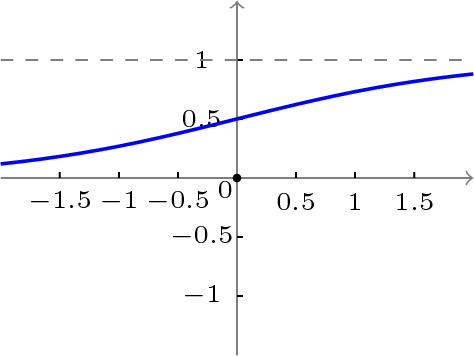双极性S型函数曲线如下图所示。

f

(

x

)

=

1

−

e

−

x

1

+

e

−

x

f(x)={1-e^{-x}\over 1+e^{-x}}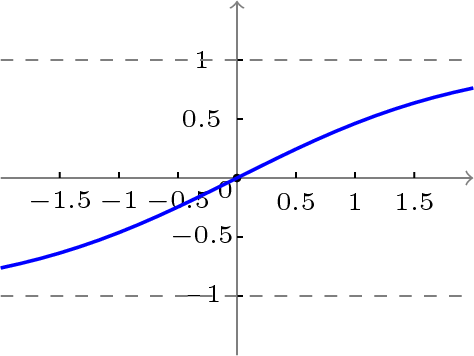3.3 BP网络的学习算法
BP网络的学习算法就是BP算法，又叫 δ 算法（在ANN的学习过程中我们会发现不少具有多个名称的术语）， 以三层感知器为例，当网络输出与期望输出不等时，存在输出误差 E ，定义如下

E

=

1

2

(

d

−

O

)

2

=

1

2

∑

κ

=

1

ℓ

(

d

k

−

o

k

)

2

E={1\over 2}(d−O)^2={1\over 2}∑_{κ=1}^ℓ(d_k−o_k)^2

将以上误差定义式展开至隐层，有

E

=

1

2

∑

κ

=

1

ℓ

[

d

κ

−

f

(

n

e

t

κ

)

]

2

=

1

2

∑

κ

=

1

ℓ

[

d

κ

−

f

(

∑

j

=

0

m

ω

j

κ

y

j

)

]

2

E={1\over 2}∑_{κ=1}^ℓ[d_κ−f(net_κ)]^2={1\over 2}∑_{κ=1}^ℓ[d_κ−f(∑_{j=0}^mω_{jκ}y_j)]^2

进一步展开至输入层，有

E

=

1

2

∑

κ

=

1

ℓ

d

κ

−

f

[

∑

j

=

0

m

ω

j

κ

f

(

n

e

t

j

)

]

2

=

1

2

∑

κ

=

1

ℓ

d

κ

−

f

[

∑

j

=

0

m

ω

j

κ

f

(

∑

j

=

0

n

υ

i

j

χ

i

)

]

2

E={1\over 2}∑_{κ=1}^ℓ{d_κ−f[∑_{j=0}^mω_{jκ}f(net_j)]}^2={1\over 2}∑_{κ=1}^ℓ{d_κ−f[∑_{j=0}^mω_{jκ}f(∑_{j=0}^nυ_{ij}χ_i)]}^2

由上式可以看出，网络输入误差是各层权值

ω

j

κ

ω_{jκ}

、

υ

i

j

υ_{ij}

的函数，因此调整权值可改变误差

E

E

。 显然，调整权值的原则是使误差不断减小，因此应使权值与误差的梯度下降成正比，即

Δ

ω

j

κ

=

−

η

∂

E

∂

ω

j

κ

j

=

0

,

1

,

2

,

…

,

m

;

κ

=

1

,

2

,

…

,

ℓ

Δω_{jκ}=−η{∂E\over ∂ω_{jκ}}j=0,1,2,…,m;κ=1,2,…,ℓ

Δ

υ

i

j

=

−

η

∂

E

∂

υ

i

j

i

=

0

,

1

,

2

,

…

,

n

;

j

=

1

,

2

,

…

,

m

Δυ_{ij}=−η{∂E∂\over υ_{ij}}i=0,1,2,…,n;j=1,2,…,m

对于一般多层感知器，设共有

h

h

个隐层，按前向顺序各隐层节点数分别记为

m

1

,

m

2

,

…

,

m

h

m_1,m_2,…,m_h

，各隐层输出分别记为

y

1

,

y

2

,

…

,

y

h

y_1,y_2,…,y_h

，各层权值矩阵分别记为

W

1

,

W

2

,

…

,

W

h

,

W

h

+

1

W_1,W_2,…,W_h,W_{h+1}

，则各层权值调整公式为
输出层

Δ

ω

j

κ

h

+

1

=

η

δ

h

+

1

κ

y

j

h

=

η

(

d

κ

−

o

κ

)

o

κ

(

1

−

o

κ

)

y

j

κ

(

j=0,1,2,

…

,mh;κ=1,2,

…

,ℓ

)

Δω^{h+1}_{jκ}=ηδ_{h+1}^κy^h_j=η(d_κ−o_κ)o_κ(1−o_κ)y^κ_j\tag{j=0,1,2,…,mh;κ=1,2,…,ℓ}

第

h

h

隐层

Δ

ω

i

j

h

=

η

δ

j

h

y

i

h

−

1

=

η

(

∑

κ

=

1

l

δ

κ

o

ω

j

κ

h

+

1

y

j

κ

(

1

−

y

j

k

a

p

p

a

)

y

i

h

−

1

(

i=0,1,2,

…

,m(h−1);j=1,2,

…

,

m

h

)

Δω^h_{ij}=ηδ^h_jy^h_i−1=η(∑^l_{κ=1}δ^o_κω^{h+1}_{jκ}y^κ_j(1−y^k_jappa)y^h_i−1\tag{i=0,1,2,…,m(h−1);j=1,2,…,$m_h$}

按以上规律逐层类推，则第一隐层权值调整公式

Δ

ω

p

q

1

=

η

δ

q

1

χ

p

=

η

(

∑

r

=

1

m

2

δ

r

2

ω

q

r

2

)

y

q

1

(

1

−

y

q

1

)

χ

p

(

p=0,1,2,

…

,n;j=1,2,

…

,

m

1

)

Δω^1_{pq}=ηδ^1_qχ_p=η(∑^{m_2}_{r=1}δ^2_rω^2_{qr})y^1_q(1−y^1_q)χ_p\tag{p=0,1,2,…,n;j=1,2,…,$m_1$}

容易看出，BP学习算法中，各层权值调整公式形式上都是一样的，均由3个因素决定，即：
学习率

η

η

本层输出的误差信号

δ

δ

本层输入信号

Y

Y

（或

X

X

）
其中输入层误差信号与网络的期望输出与实际输出之差有关，直接反应了输出误差，而各隐层的误差信号与前面各层的误差信号有关，是从输出层开始逐层反传过来的。
可以看出BP算法属于δ学习规则类，这类算法常被称为误差的梯度下降算法。δ学习规则可以看成是Widrow-Hoff(LMS)学习规则的一般化(generalize)情况。LMS学习规则与神经元采用的变换函数无关，因而不需要对变换函数求导，δ学习规则则没有这个性质，要求变换函数可导。这就是为什么我们前面采用Sigmoid函数的原因。
综上所述，BP三要素如下图所示。下面我们会介绍BP网络的学习训练的具体过程。
BP网络的训练分解
训练一个BP神经网络，实际上就是调整网络的权重和偏置这两个参数，BP神经网络的训练过程分两部分：
前向传输，逐层波浪式的传递输出值；逆向反馈，反向逐层调整权重和偏置； 我们先来看前向传输。
前向传输（Feed-Forward前向反馈）
在训练网络之前，我们需要随机初始化权重和偏置，对每一个权重取

[

−

1

,

1

]

[-1,1]

的一个随机实数，每一个偏置取

[

0

,

1

]

[0,1]

的一个随机实数，之后就开始进行前向传输。
神经网络的训练是由多趟迭代完成的，每一趟迭代都使用训练集的所有记录，而每一次训练网络只使用一条记录，抽象的描述如下：
while 终止条件未满足：
for record:dataset:
trainModel(record)

首先设置输入层的输出值，假设属性的个数为100，那我们就设置输入层的神经单元个数为100，输入层的结点

N

i

N_i

为记录第

i

i

维上的属性值

x

i

x_i

。对输入层的操作就这么简单，之后的每层就要复杂一些了，除输入层外，其他各层的输入值是上一层输入值按权重累加的结果值加上偏置，每个结点的输出值等该结点的输入值作变换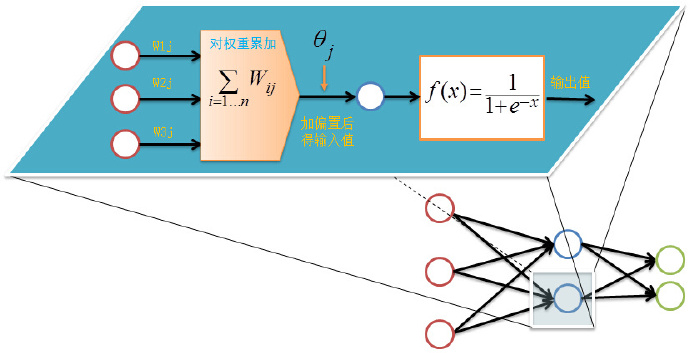前向传输的输出层的计算过程公式如下：

I

j

=

∑

i

=

1

ω

i

j

o

i

+

θ

j

I_j=\sum_{i=1}\omega_{ij}o_i+\theta_j

o

j

=

f

(

I

j

)

=

1

1

+

e

I

j

o_j=f(I_j)={1\over 1+e^{I_j}}

对隐藏层和输出层的每一个结点都按照如上图的方式计算输出值，就完成前向传播的过程，紧接着是进行逆向反馈。
逆向反馈（Backpropagation）
逆向反馈从最后一层即输出层开始，我们训练神经网络作分类的目的往往是希望最后一层的输出能够描述数据记录的类别，比如对于一个二分类的问题，我们常常用两个神经单元作为输出层，如果输出层的第一个神经单元的输出值比第二个神经单元大，我们认为这个数据记录属于第一类，否则属于第二类。
还记得我们第一次前向反馈时，整个网络的权重和偏置都是我们随机取，因此网络的输出肯定还不能描述记录的类别，因此需要调整网络的参数，即权重值和偏置值，而调整的依据就是网络的输出层的输出值与类别之间的差异，通过调整参数来缩小这个差异，这就是神经网络的优化目标。对于输出层：

E

j

=

O

j

(

1

−

O

j

)

(

T

j

−

O

j

)

E_j=O_j(1-O_j)(T_j-O_j)

其中

E

j

E_j

表示第

j

j

个结点的误差值，

O

j

O_j

表示第

j

j

个结点的输出值，

T

j

T_j

记录输出值，比如对于2分类问题，我们用01表示类标1,10表示类别2，如果一个记录属于类别1，那么其

T

1

=

0

T_1=0

，

T

2

=

1

T_2=1

。
中间的隐藏层并不直接与数据记录的类别打交道，而是通过下一层的所有结点误差按权重累加，计算公式如下：

E

j

=

O

j

(

1

−

O

j

)

∑

k

E

k

W

j

k

E_j=O_j(1-O_j)\sum_kE_kW_{jk}

其中

W

j

k

W_{jk}

表示当前层的结点

j

j

到下一层的结点

k

k

的权重值，

E

k

E_k

下一层的结点

k

k

的误差率。
计算完误差率后，就可以利用误差率对权重和偏置进行更新，首先看权重的更新：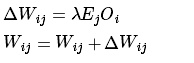其中

λ

λ

表示表示学习速率，取值为0到1，学习速率设置得大，训练收敛更快，但容易陷入局部最优解，学习速率设置得比较小的话，收敛速度较慢，但能一步步逼近全局最优解。
更新完权重后，还有最后一项参数需要更新，即偏置：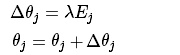至此，我们完成了一次神经网络的训练过程，通过不断的使用所有数据记录进行训练，从而得到一个分类模型。不断地迭代，不可能无休止的下去，总归有个终止条件。
训练终止条件
每一轮训练都使用数据集的所有记录，但什么时候停止，停止条件有下面两种：
设置最大迭代次数，比如使用数据集迭代100次后停止训练计算训练集在网络上的预测准确率，达到一定门限值后停止训练
BP网络运行的具体流程
网络结构
输入层有

n

n

个神经元，隐含层有

p

p

个神经元，输出层有

q

q

个神经元。
变量定义
输入变量：

x

=

(

x

1

,

x

2

,

…

,

x

n

)

x=(x_1,x_2,\dots,x_n)

隐含层输入变量：

h

i

=

(

h

i

1

,

h

i

2

,

…

,

h

i

p

)

hi=(hi_1,hi_2,\dots,hi_p)

隐含层输出变量：

h

o

=

(

h

o

1

,

h

o

2

,

…

,

h

o

p

)

ho=(ho_1,ho_2,\dots,ho_p)

输出层输入变量：

y

i

=

(

y

i

1

,

y

i

2

,

…

,

y

i

q

)

yi=(yi_1,yi_2,\dots,yi_q)

输出层输出变量：

y

o

=

(

y

o

1

,

y

o

2

,

…

,

y

o

q

)

yo=(yo_1,yo_2,\dots,yo_q)

期望输出向量：

d

o

=

(

d

1

,

d

2

,

…

,

d

q

)

d_o=(d_1,d_2,\dots,d_q)

输入层与中间层的连接权值：

w

i

h

w_{ih}

隐含层与输出层的连接权值：

w

h

o

w_{ho}

隐含层各神经元的阈值：

b

h

b_h

输出层各神经元的阈值：

b

o

b_o

样本数据个数：

k

=

1

,

2

,

…

,

m

k=1,2,\dots,m

激活函数：

f

(

⋅

)

f(\cdot)

误差函数：

e

=

1

2

∑

o

=

1

q

(

d

o

(

k

)

−

y

o

o

(

k

)

)

2

e={1\over 2}\sum_{o=1}^{q}(d_o(k)-yo_o(k))^2

###第一步：网络初始化 给各连接权值分别赋一个区间

(

−

1

,

1

)

(-1,1)

内的随机数，设定误差函数

e

e

，给定计算精度值

ε

\varepsilon

和最大学习次数

M

M

。 ###第二步：随机选取 随机选取第

k

k

个输入样本以及对应的期望输出

x

(

k

)

=

(

x

1

(

k

)

,

x

2

(

k

)

,

…

,

x

n

(

k

)

)

d

o

(

k

)

=

(

d

1

(

k

)

,

d

2

(

k

)

,

…

,

d

q

(

k

)

)

x(k)=(x_1(k),x_2(k),\dots,x_n(k))\\ d_o(k)=(d_1(k),d_2(k),\dots,d_q(k))

###第三部：隐含层计算 计算隐含层各神经元的输入和输出

h

i

h

(

k

)

=

∑

i

=

1

n

w

i

h

x

i

(

k

)

−

b

h

(

h

=

1

,

2

,

…

,

p

)

hi_h(k)=\sum_{i=1}^nw_{ih}x_i(k)-b_h \tag{$h=1,2,\dots,p$}

h

i

h

(

k

)

=

f

(

h

i

h

(

k

)

)

(

h

=

1

,

2

,

…

,

p

)

hi_h(k)=f(hi_h(k)) \tag{$h=1,2,\dots,p$}

y

i

o

(

k

)

=

∑

h

=

1

p

w

h

o

h

o

h

(

k

)

−

b

o

(

o=1,2,

…

,q

)

yi_o(k)=\sum_{h=1}^pw_{ho}ho_h(k)-b_o \tag{o=1,2,$\dots$,q}

y

o

o

(

k

)

=

f

(

y

i

o

(

k

)

)

(

o=1,2,

…

,q

)

yo_o(k)=f(yi_o(k))\tag{o=1,2,$\dots$,q}

第四步：求偏导数
利用网络期望输出和实际输出，计算误差函数对输出层的各神经元的偏导数

δ

o

(

k

)

\delta_o(k)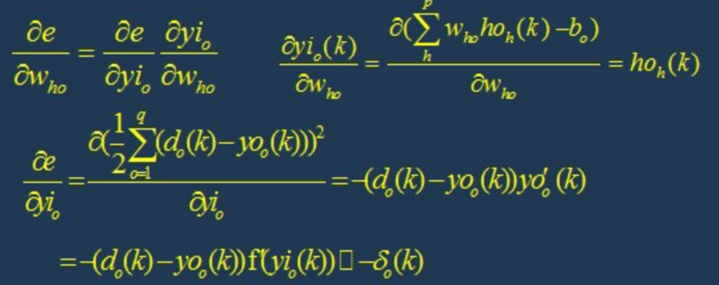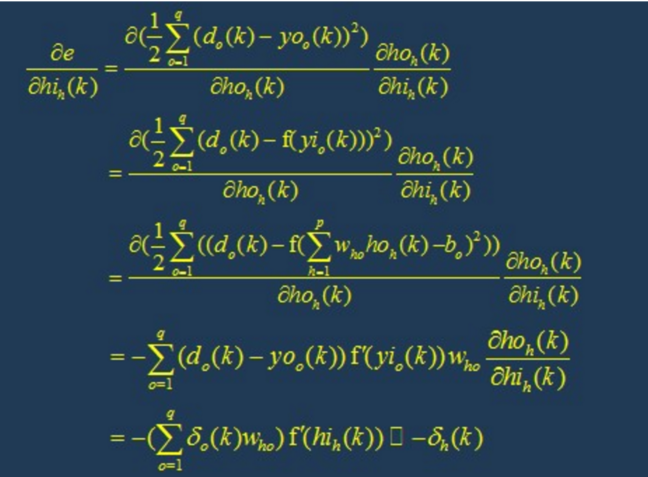第六步：修正权值
利用输出层各神经元的

δ

o

(

k

)

\delta_o(k)

和隐含层各神经元的输出来修正连接权值

w

h

o

(

k

)

w_{ho}(k)

。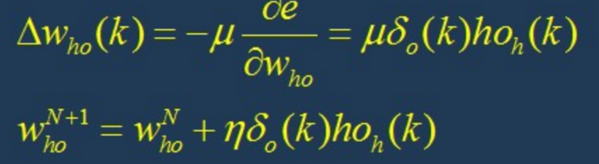第七部：修正权值
利用隐含层各神经元的

δ

h

(

k

)

\delta_h(k)

和输入层各神经元的输入修正连接权值。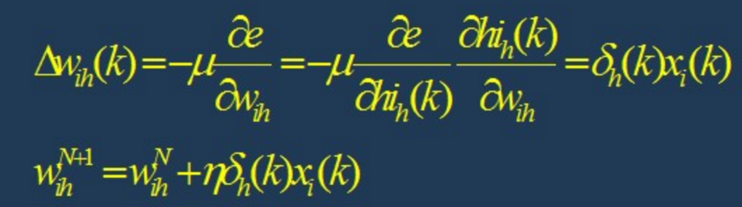第八步：计算全局误差

E

=

1

2

m

∑

k

=

1

m

∑

o

=

1

q

(

d

o

(

k

)

−

y

o

(

k

)

)

2

E={1\over 2m}\sum_{k=1}^m\sum_{o=1}^q(d_o(k)-y_o(k))^2

第九步：判断模型合理性
判断网络误差是否满足要求。 当误差达到预设精度或者学习次数大于设计的最大次数，则结束算法。 否则，选取下一个学习样本以及对应的输出期望，返回第三部，进入下一轮学习。
BP网络的设计
在进行BP网络的设计是，一般应从网络的层数、每层中的神经元个数和激活函数、初始值以及学习速率等几个方面来进行考虑，下面是一些选取的原则。
1.网络的层数
理论已经证明，具有偏差和至少一个S型隐层加上一个线性输出层的网络，能够逼近任何有理函数，增加层数可以进一步降低误差，提高精度，但同时也是网络 复杂化。另外不能用仅具有非线性激活函数的单层网络来解决问题，因为能用单层网络解决的问题，用自适应线性网络也一定能解决，而且自适应线性网络的 运算速度更快，而对于只能用非线性函数解决的问题，单层精度又不够高，也只有增加层数才能达到期望的结果。
2.隐层神经元的个数
网络训练精度的提高，可以通过采用一个隐含层，而增加其神经元个数的方法来获得，这在结构实现上要比增加网络层数简单得多。一般而言，我们用精度和 训练网络的时间来恒量一个神经网络设计的好坏： （1）神经元数太少时，网络不能很好的学习，训练迭代的次数也比较多，训练精度也不高。 （2）神经元数太多时，网络的功能越强大，精确度也更高，训练迭代的次数也大，可能会出现过拟合(over fitting)现象。 由此，我们得到神经网络隐层神经元个数的选取原则是：在能够解决问题的前提下，再加上一两个神经元，以加快误差下降速度即可。
3.初始权值的选取
一般初始权值是取值在(−1,1)之间的随机数。另外威得罗等人在分析了两层网络是如何对一个函数进行训练后，提出选择初始权值量级为s√r的策略， 其中r为输入个数，s为第一层神经元个数。
4.学习速率
学习速率一般选取为0.01−0.8，大的学习速率可能导致系统的不稳定，但小的学习速率导致收敛太慢，需要较长的训练时间。对于较复杂的网络， 在误差曲面的不同位置可能需要不同的学习速率，为了减少寻找学习速率的训练次数及时间，比较合适的方法是采用变化的自适应学习速率，使网络在 不同的阶段设置不同大小的学习速率。
5.期望误差的选取
在设计网络的过程中，期望误差值也应当通过对比训练后确定一个合适的值，这个合适的值是相对于所需要的隐层节点数来确定的。一般情况下，可以同时对两个不同 的期望误差值的网络进行训练，最后通过综合因素来确定其中一个网络。
BP网络的局限性
BP网络具有以下的几个问题：
(1)需要较长的训练时间：这主要是由于学习速率太小所造成的，可采用变化的或自适应的学习速率来加以改进。(2)完全不能训练：这主要表现在网络的麻痹上，通常为了避免这种情况的产生，一是选取较小的初始权值，而是采用较小的学习速率。(3)局部最小值：这里采用的梯度下降法可能收敛到局部最小值，采用多层网络或较多的神经元，有可能得到更好的结果。 BP网络的改进

P算法改进的主要目标是加快训练速度，避免陷入局部极小值等，常见的改进方法有带动量因子算法、自适应学习速率、变化的学习速率以及作用函数后缩法等。 动量因子法的基本思想是在反向传播的基础上，在每一个权值的变化上加上一项正比于前次权值变化的值，并根据反向传播法来产生新的权值变化。而自适应学习 速率的方法则是针对一些特定的问题的。改变学习速率的方法的原则是，若连续几次迭代中，若目标函数对某个权倒数的符号相同，则这个权的学习速率增加， 反之若符号相反则减小它的学习速率。而作用函数后缩法则是将作用函数进行平移，即加上一个常数。
BP网络实现
由于BP网络具有出色的非线性映射能力、泛化能力和容错能力，因此BP网络成了至今为止应用最广泛的人工神经网络。下图是Matlab下用BP网络做线性拟合的结果，效果很好。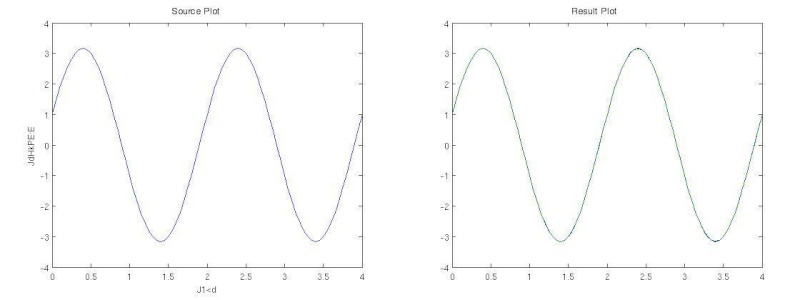% BP网络函数逼近实例
% 1.首先定义正弦函数，采样率为20Hz，频率为1Hz
k = 1; % 设定正弦信号频率
p = [0:0.05:4];
t = cos(k*pi*p) + 3*sin(pi*p);
plot(p, t, '-'), xlabel('时间'); ylabel('输入信号');
% 2.生成BP网络。用newff函数生成前向型BP网络，设定隐层中神经元数目为10
% 分别选择隐层的传递函数为 tansig，输出层的传递函数为 purelin，
% 学习算法为trainlm。
net =
newff(minmax(p),[10,10,1],{'tansig','tansig','purelin'},'trainlm');
% 3.对生成的网络进行仿真并做图显示。
y1 = sim(net,p); plot(p, t, '-', p, y1, '--')
% 4.训练。对网络进行训练，设定训练误差目标为 1e-5，最大迭代次数为300，
% 学习速率为0.05。
net.trainParam.lr=0.05;
net.trainParam.epochs=1000;
net.trainParam.goal=1e-5;
[net,tr]=train(net,p,t);
%5.再次对生成的网络进行仿真并做图显示。
y2 = sim(net,p);
plot(p, t, '-', p, y2, '--')

这是用C语言写的：用BP神经网络拟合函数：

Y

=

s

i

n

(

X

)

Y=sin(X)

#include "math.h"
#include "time.h"
#include "stdio.h"
#include "stdlib.h"
#include "ctype.h"
#define Ni 1
#define Nm 4
#define No 1
#define L 100
#define Enom 0.02
#define loopmax 100000
#define e 2.71828
double E;
double a,u,n;
double W1[Ni][Nm],D1[Ni][Nm],W2[Nm][No],D2[Nm][No];
double D22[Nm][No],D11[Ni][No];
double a1[Ni][Nm],a2[Nm][No];
double Pi[L][Ni],Pm[L][Nm],Po[L][No],T[L][No];
double Xm[L][Nm],Xo[L][No];
double Qm[L][Nm],Qo[L][No];
void proceed();
void proceedR();
void forQ();
void amend();
void initiate();
double newa(double a,double D);
double cal(double d);
double vcal(double d);
main()
{
long int i;
int flag;
char choice;
for(;;)
{
flag=0;
initiate();
for(i=0;;i++)
{
proceed();
if( E < Enom )
{
flag=1;
break;
}
if( i >= loopmax)
{
flag = -1;
break;
}
if(i%2500==0)
printf("第%10d轮误差：%20f,学习速率:%10f\n",i,E,a1);
forQ();
amend();
}
if(flag>0)proceedR();
else printf("训练失败！\n");
for(;;)
{
choice=getchar();
printf("是否继续？(Y/N)\n");
choice=getchar();
choice=toupper(choice);
if(choice=='Y')break;
if(choice=='N')exit(0);
}
}
}
void initiate()
{
int i,j;
int random;
double x;
double step;
int stime;
long ltime;
ltime=time(NULL);
stime=(unsigned)ltime/2;
srand(stime);
a=0.02;
u=1;
n=1;
printf("本程序将用BP神经网络拟合函数：Y=sin(X)\n\n");
for( i=0; i<Nm; i++)
{
for( j=0; j<Ni; j++)
{
random=rand()%100-50;
x=random;
x=x/100;
W1[j][i]=x;
D11[j][i]=0;
D1[j][i]=0;
a1[j][i]=0.01;
}
for( j=0; j<No; j++)
{
random=rand()%100-50;
x=random;
x=x/100;
W2[i][j]=x;
D22[i][j]=0;
D2[i][j]=0;
a2[i][j]=0.01;
}
}
step=1.0/L;
for(i=0;i<L;i++)
{
x=i;
Pi[i]=x*step;
T[i]=sin(Pi[i]);
}
printf("初始化成功!\n\n下面将对神经网络进行训练请稍候。\n");
}
void proceed()
{
int i, j, k;
E=0 ;
for( i=0; i<L; i++ )
{
for( j=0; j<Nm; j++ )
{
Pm[i][j] = 0;
for( k=0; k<Ni; k++ )
{
Pm[i][j] = Pi[i][k] * W1[k][j] + Pm[i][j];
}
Xm[i][j] = cal( Pm[i][j] );
}
for( j=0; j<No; j++)
{
Po[i][j] = 0;
for( k=0; k<Nm; k++)
{
Po[i][j] = Xm[i][k] * W2[k][j] + Po[i][j];
}
Xo[i][j] = cal( Po[i][j] );
E = E + ( Xo[i][j] - T[i][j] ) * ( Xo[i][j] - T[i][j] ) / 2;
}
}
}
void forQ()
{
int i,j,k;
for( i=0; i<L; i++ )
{
for( j=0; j<No; j++)
{
Qo[i][j] = ( T[i][j] - Xo[i][j] )* vcal( Xo[i][j] );
}
for(j=0; j<Nm; j++)
{
Qm[i][j]=0;
for( k=0; k<No; k++)
{
Qm[i][j] = Qo[i][k] * W2[j][k] + Qm[i][j];
}
Qm[i][j] = Qm[i][j] * vcal( Xm[i][j] );
}
}
}
void amend()
{
int i,j,k;
double D;
for( i=0; i<Nm; i++)
{
for( j=0; j<Ni; j++)
{
D1[j][i]=0;
}
for( j=0; j<No; j++)
{
D2[i][j]=0;
}
}
for( i=0; i<Ni; i++)
{
for( j=0; j<Nm; j++)
{
for( k=0; k<L; k++)
{
D1[i][j] = Qm[k][j] * Pi[k][i] + D1[i][j];
}
D = D1[i][j] * D11[i][j]  ;//为D11付初值
a1[i][j] = newa( a1[i][j] , D );  // a 付初值
W1[i][j] = W1[i][j] + a1[i][j] * ( n * D1[i][j] + ( 1 - n ) * D11[i][j] );
D11[i][j] = D1[i][j];
}
}
for( i=0; i<Nm; i++)
{
for( j=0; j<No; j++)
{
for( k=0; k<L; k++)
{
D2[i][j] = Qo[k][j] * Xm[k][i] + D2[i][j];
}
D = D2[i][j] * D22[i][j]  ;//为D11付初值
a2[i][j] = newa( a2[i][j] , D );
W2[i][j] = W2[i][j] + a2[i][j] * ( n * D2[i][j] + ( 1 - n ) * D22[i][j] );
D22[i][j] = D2[i][j];
}
}
}
void proceedR()
{
int i, j;
float x;
double input,output;
char choice;
for(;;)
{
for(;;)
{
printf("在此输入需要计算的值(0,1):\n");
scanf("%f",&x);
input=(double)x;
if((input>=0)&(input<=1))break;
printf("注意输入值应介于0、1之间！\n");
for(;;)
{
choice=getchar();
printf("是否继续？(Y/N)\n");
choice=getchar();
choice=toupper(choice);
if(choice=='Y')break;
if(choice=='N')exit(0);
}
}
for(i=0;i<Nm;i++)
{
Pm[i]=0;
for( j=0; j<Ni; j++ )
{
Pm[i] =  input* W1[j][i]+Pm[i] ;
}
Xm[i] = cal( Pm[i] );
}
for( i=0; i<No; i++)
{
Po[i] = 0;
for( j=0; j<Nm; j++)
{
Po[i] = Xm[j] * W2[j][i]+Po[i];
}
}
output=cal( Po );
printf("输入值为%20f对应的结果为%f\n",input,output);
printf("输入值为%20f对应的正常结果为%f\n",input,sin(input));
for(;;)
{
choice=getchar();
printf("是否继续？(Y/N)\n");
choice=getchar();
choice=toupper(choice);
if(choice=='Y')break;
if(choice=='N')exit(0);
}
}
}

double newa(double a, double D)
{
if( D > 0 )
{
{
if(a<=0.04)
a = a * 2;
else a=0.08;
}
}
else
if ( D < 0)
{
if(a>=0.02)
{
a = a / 2;
}
else a=0.01;
}
return a;
}
double cal(double d)
{
d =  - (d * u);                                //              chushihua
d = exp( d );
d = 1 / ( 1 + d );
return d;
}
double vcal(double d)
{
return u * d * ( 1 - d );
}


展开全文• 合理的在线购物行为被定义为“由于虚拟网络环境中各种因素的影响，消费者做出的一系列不合理的决策行为”。 本文旨在探讨心理会计影响下的过度消费，冲动性消费和捆绑消费的非理性网购行为，并从心理会计理论的...
• 常见网络命令的使用 实验目的理解验证常用网络命令的原理和功能掌握常用的网络命令使用方法合理使用相关命令对网络进行管理与维护 实验内容 网络参数查询命令IPCONFIG和IP地址相关计算 网络测试命令Ping 命令和网络...
• ## ucinet网络分析使用总结

万次阅读 多人点赞 2019-08-20 17:10:32
UCINET为菜单驱动的Windows程序，可能是最知名和最经常被使用的处理社会网络数据和其他相似性数据的综合性分析程序。与UCINET捆绑在一 起的还有Pajek、Mage和NetDraw等三个软件。UCINET能够处理的原始数据为矩阵格式...
ucinet介绍
UCINET为菜单驱动的Windows程序，可能是最知名和最经常被使用的处理社会网络数据和其他相似性数据的综合性分析程序。与UCINET捆绑在一起的还有Pajek、Mage和NetDraw等三个软件。UCINET能够处理的原始数据为矩阵格式，提供了大量数据管理和转化工具。该程序本身不包含 网络可视化的图形程序，但可将数据和处理结果输出至NetDraw、Pajek、Mage和KrackPlot等软件作图。UCINET包含大量包括探测凝聚子群（cliques, clans, plexes）和区域（components, cores）、中心性分析（centrality）、个人网络分析和结构洞分析在内的网络分析程序。UCINET还包含为数众多的基于过程的分析程序，如聚类分析、多维标度、二模标度（奇异值分解、因子分析和对应分析）、角色和地位分析（结构、角色和正则对等性）和拟合中心-边缘模型。此外，UCINET提供了从简单统计到拟合p1模型在内的多种统计程序。
ucinet使用技巧
ucinet的安装我觉得不用多说，因为不论是csdn本站，还是百度里面都有很多的资源可以下载，一般都是英文版，所以我就不从开头介绍了。另外就是虽然很多东西当初用过并且记得，但等过了一段时间后，再次看这个功能其实很可能英文单词的意思都已经忘了，就记得如何去使用。所以这里，我直接以一个案例的方式进行演示：
1.数据导入： Ucinet的数据输入方式有多种:初始数据(Raw)、Excel数据和数据语言数据(Data Language，DL)。Ucinet处理的Excel数据最多只能有255列。就好像我记得excel的最大存储数据为104万左右，超过就会提示显示不全。ucinet它导入数据步骤为输入路径:数据>输入> Excel矩阵：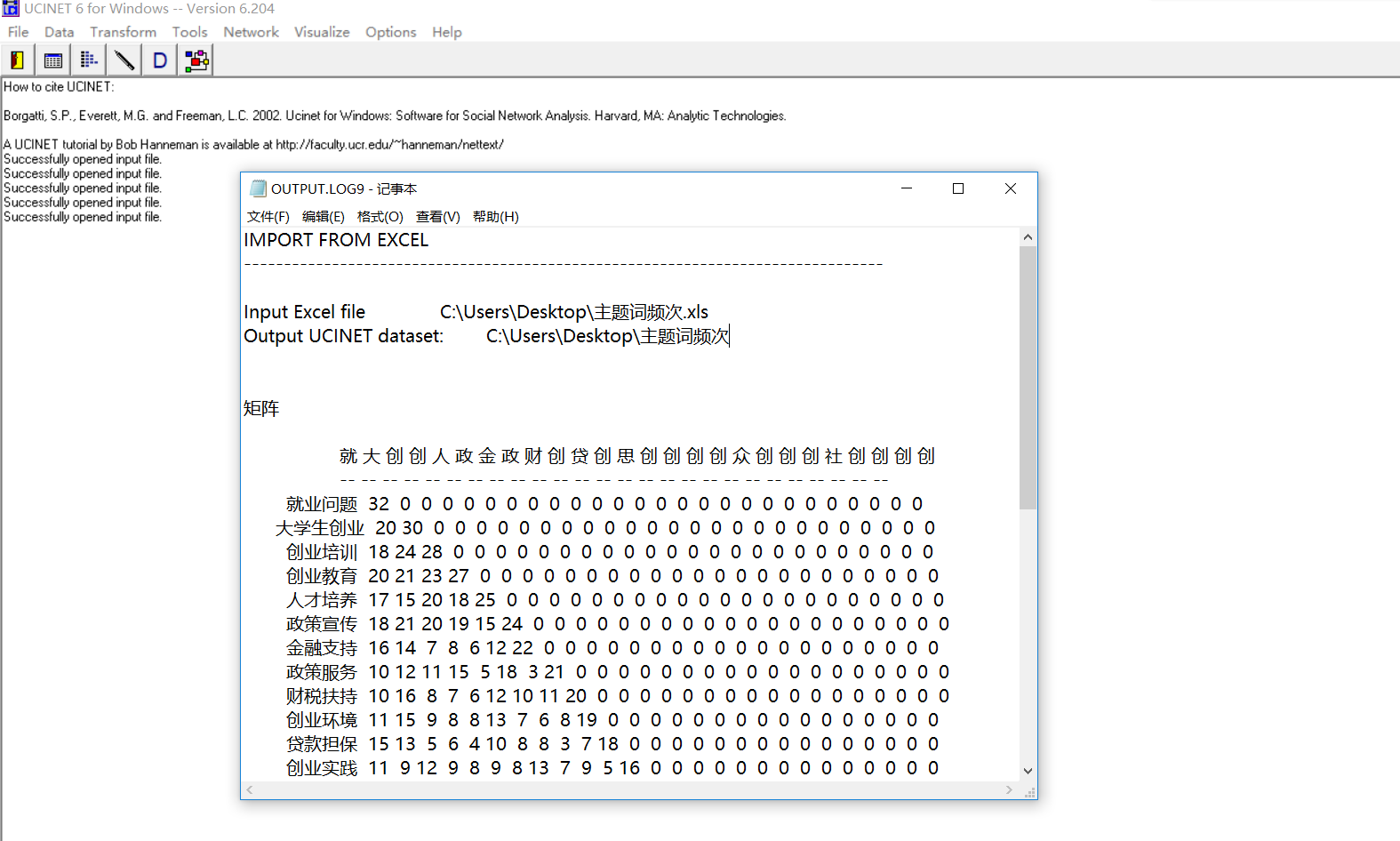当然，这里只是为了测试是否这个数据可以被ucinet所引用，不会有什么乱码或者其它格式问题，另外如果中途选择了什么其它操作，那么除了上述的打印外，还将生成了一个新的excel文件位于上面的路径。

2.可视化数据分析
网络密度指的是网络中各个成员之间联系的紧密度，可以通过网络中实际存在的关系数与理论上可能存在的关系数相比得到，成员之间的联系越多，该网络的密度越大。整体网的密度越大，该网络对其中行动者的态度、行为等产生的影响可能越大。计算的时候最好将多值关系数据转换成二值关系数据。将多值关系数据转换成二值关系数据路径:变换→对分。
在对原始数据进行二值化处理以后，所有的权值变为了0和1（即是否有关联），这样计算出来的结果是普通的中心度。想要得到加权网络的中心性，只需要在矩阵数据中保留权值，不进行二值化计算即可，和之前的唯一区别在于计算公式中的0与1变成了具体的权值。
我们这里也可以进行二值化，将上述的频次转换成只有0、1变量的数据，我这里不转了，依据的公式一般是：

H

i

j

=

{

1,

&ThinSpace;&ThinSpace;

if
&ThinSpace;&ThinSpace;

x

i

∈

N

k

(

x

j

)

&ThinSpace;&ThinSpace;

or
&ThinSpace;&ThinSpace;

x

j

∈

N

k

(

x

i

)

0,

&ThinSpace;&ThinSpace;

otherwise
&ThinSpace;&ThinSpace;

}

H_{ij}=\left\{ \begin{matrix} \text{1,}&amp; \,\,\text{if\,\,}x_i\in N_k\left( x_j \right) \quad \,\,\text{or\,\,}x_j\in N_k\left( x_i \right)\\ \text{0,}&amp; \,\,\text{otherwise\,\,}\\ \end{matrix} \right\}

其中

N

k

(

x

i

)

N_k\left( x_i \right)

表示数据集中距离

x

i

x_{i}

最近的 k 个邻居的集合。
然后我们需要将excel文件转换成ucinet能识别的类型，即.h结尾的文件，这里有很多种转换的方式，我比较喜欢的是打开一个矩阵，然后将数据导入，这里它会提示imported success，然后将其以.h文件保存到桌面，然后就可以开始可视化了。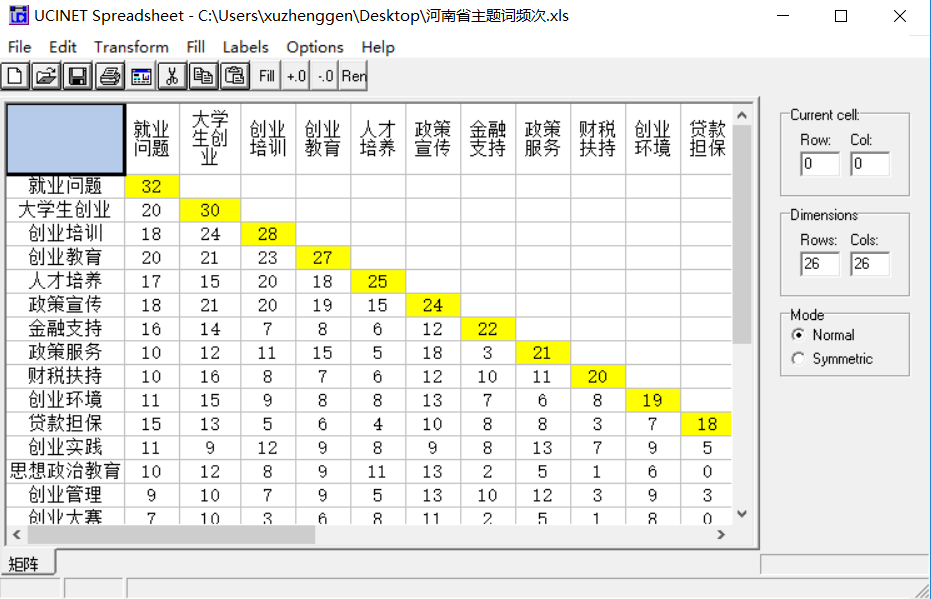最后进入netdraw直接可视化，选项为Net- Draw >Open > Ucinet Dataset > Network：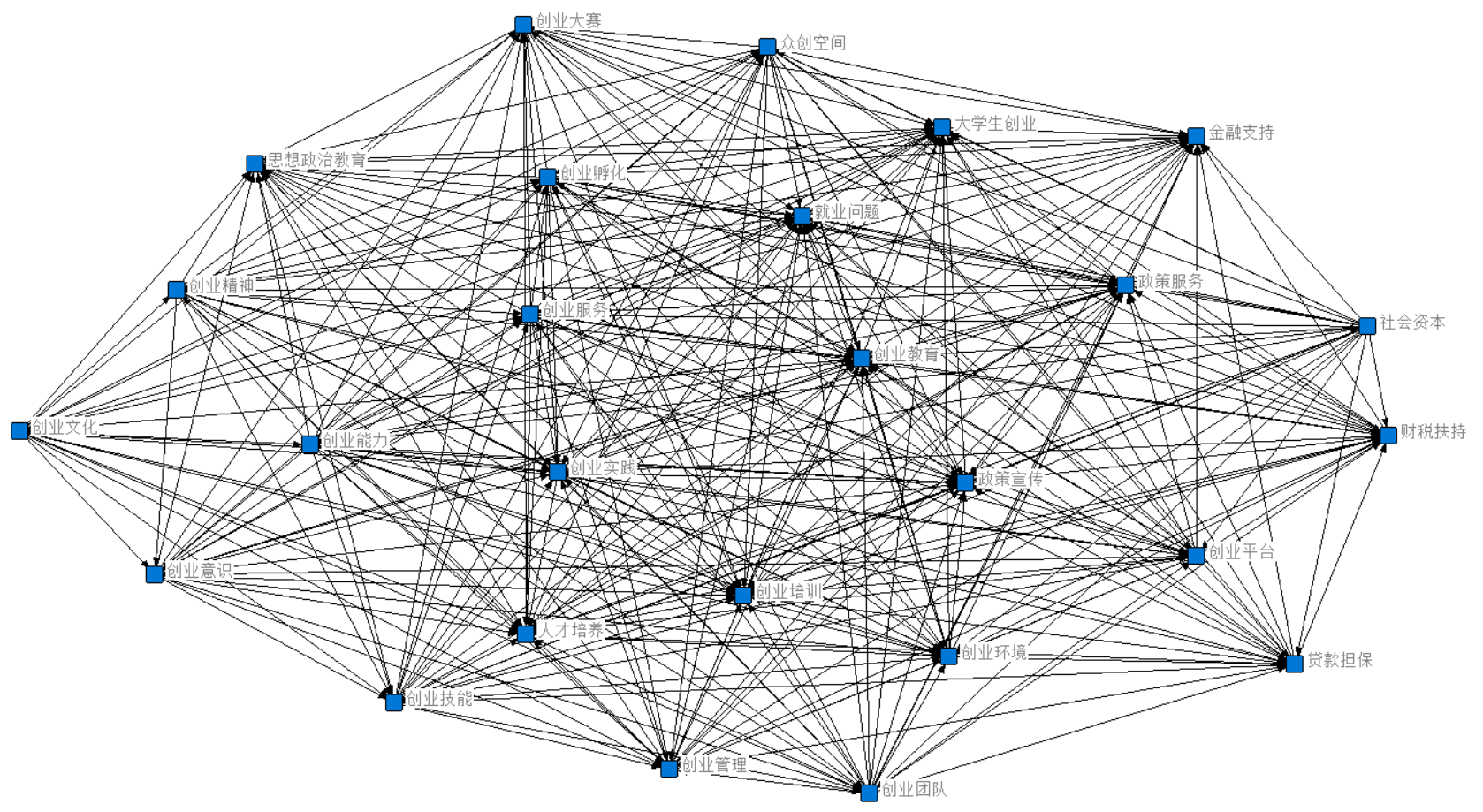这里我们可以在右边栏看到可选框，可以根据喜好改变点的大小颜色以及线的粗细，另外就是去除某个点对整体图的影响，检测整个系统的波动性和稳定性，这个我记得我之前都是做层次分析法或者PCA用的，但在网络里自己也没试过这个。
我主要试的是图上面的工具栏，可以选择中心性的网络可视化，即centrality measures：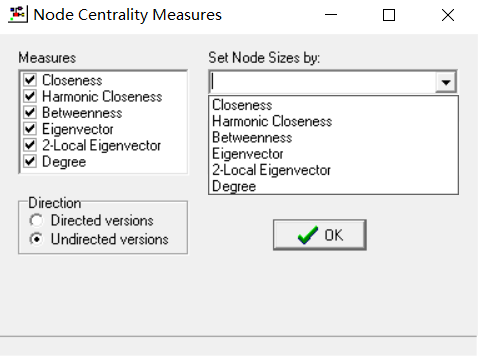选择任何一个图都会根据其改变，比如我选择closeness：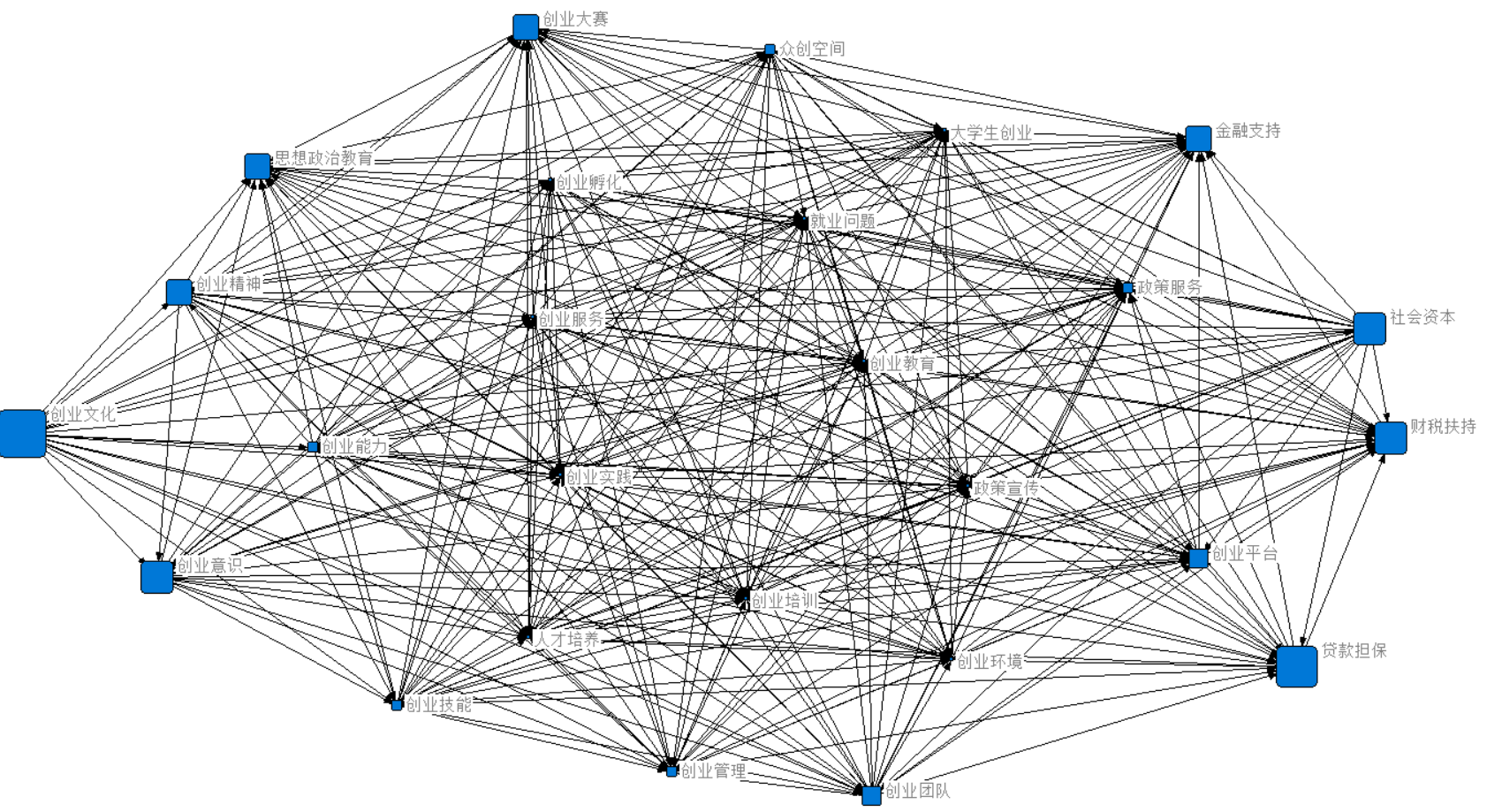然后关于上述的区别，也就是我接下来要总结的，但只是做一个粗略总结。
3、社会网络分析技术
首先如果数据不是非结构化数据，并且它的缺失值与异常值都在合理范围内（关于上述两者的概念，我在另一篇博客有提：kaggle（一）：随机森林与泰坦尼克），那么我们可以做一个相关性分析，相关系数公式即为：

p

i

j

=

E

(

y

i

y

j

)

−

E

(

y

i

)

E

(

y

j

)

(

[

E

(

y

i

2

)

−

(

E

(

y

i

)

)

2

]

⋅

[

E

(

y

j

2

)

−

(

E

(

y

j

)

)

2

]

p_{ij}=\frac{E\left( y_iy_j \right) -E\left( y_i \right) E\left( y_j \right)}{\sqrt{\left( \left[ E\left( y_{i}^{2} \right) -\left( E\left( y_i \right) \right) ^2 \right] \cdot \left[ E\left( y_{j}^{2} \right) -\left( E\left( y_j \right) \right) ^2 \right] \right.}}

可以根据它得到相关系数权值矩阵，以此去判断一些指标权重密度还有啥的，不过ucinet基本都封装好了，我在这里提一下，因为我下面的数据基本都是围绕这个来弄，

社会网络分析技术主要有网络密度、中心性分析、凝聚子群分析、核心-边缘分析等，这里对涉及到的前三种方法作简单介绍。
中心性(centrality)是度量整个网络中心化程度的重要指标，在城市群网络中，处于中心位置的城市更易获得资源和信息，拥有更大的权力和对其他城市更强的影响力。网络中心性又可以分为点度中心度、接近中心度和中间中心度三个指标。
度数中心性（Degree）中间中心性（betweenness）接近中心性（Closeness）绝对点度中心度

C

A

D

i

=

d

(

i

)

=

∑

j

X

i

j

C

A

B

i

=

∑

j

&lt;

k

b

j

k

(

i

)

=

∑

j

&lt;

k

g

j

k

(

i

)

/

g

j

k

C_{A B i}=\sum_{j&lt;k} b_{j k}(i)=\sum_{j&lt;k} g_{j k}(i) / g_{j k}

C

A

P

i

−

1

=

∑

j

d

i

j

C_{A P i}^{-1}=\sum_{j} d_{i j}

相对点度中心度

C

R

D

i

=

d

(

i

)

/

(

n

−

1

)

C_{RDi}^{}=d\left( i \right) /\left( n-1 \right)

C

R

B

i

=

2

C

A

B

i

/

[

(

n

−

1

)

(

n

−

2

)

]

C_{RBi}^{}=2C_{ABi}^{}/\left[ \left( n-1 \right) \left( n-2 \right) \right]

C

R

P

i

−

1

=

2

C

A

P

i

−

1

/

(

n

−

1

)

C_{RPi}^{-1}=2C_{APi}^{-1}/\left( n-1 \right)

图的中心势

C

A

D

=

∑

i

(

C

A

D

max

⁡

−

C

A

D

i

)

(

n

−

1

)

(

n

−

2

)

C

R

D

=

∑

i

(

C

R

D

max

⁡

−

C

R

D

i

)

n

−

2

\begin{array}{l}{C_{A D}=\frac{\sum_{i}\left(C_{A D \max }-C_{A D i}\right)}{(n-1)(n-2)}} \\ {C_{R D}=\frac{\sum_{i}\left(C_{R D \max }-C_{R D i}\right)}{n-2}}\end{array}

C

B

=

2

∑

i

(

C

A

B

max

⁡

−

C

A

B

i

)

/

[

(

n

−

1

)

2

(

n

−

2

)

]

=

2

∑

i

(

C

B

B

max

⁡

−

C

R

B

i

)

/

(

n

−

1

)

C_B=2\sum_i{\left( C_{AB\max}-C_{ABi} \right)}/\left[ \left( n-1 \right) ^2\left( n-2 \right) \right] =2\sum_i{\left( C_{BB\max}-C_{RBi} \right)}/\left( n-1 \right)

C

C

=

∑

i

(

C

R

C

max

⁡

′

−

C

R

C

i

′

)

(

n

−

2

)

(

n

−

1

)

(

2

n

−

3

)

C_{C}=\frac{\sum_{i}\left(C_{R C \max }^{\prime}-C_{R C i}^{\prime}\right)}{(n-2)(n-1)}(2 n-3)

上表摘自SNA(社会网络分析)——三种中心度总结，这张表里的有些公式和我之前建模用过的差不多，不外乎就是中间有进行过变式，就比如我之前喜欢写

n

3

−

4

n

2

+

5

n

−

2

=

(

n

−

1

)

2

(

n

−

2

)

n^{3} - 4n^{2} + 5n -2 = \left( n-1 \right) ^2\left( n-2 \right)

，然后中间具体怎么推导的，例如betweenness可以看我给的参考链接2，我不是专门研究的我也不太清楚。我们还可以来看一下相关的图，对于上述三种情况的文字对比图：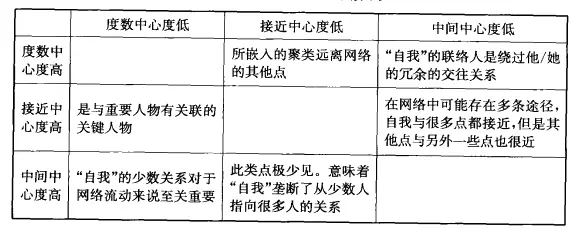然后我们就可以用ucinet软件做出上述三种关系，这里只做一个，因为步骤操作都是基本一致的，选择network ——> centrality ——> Degree ，就可以得到Degree centrality ：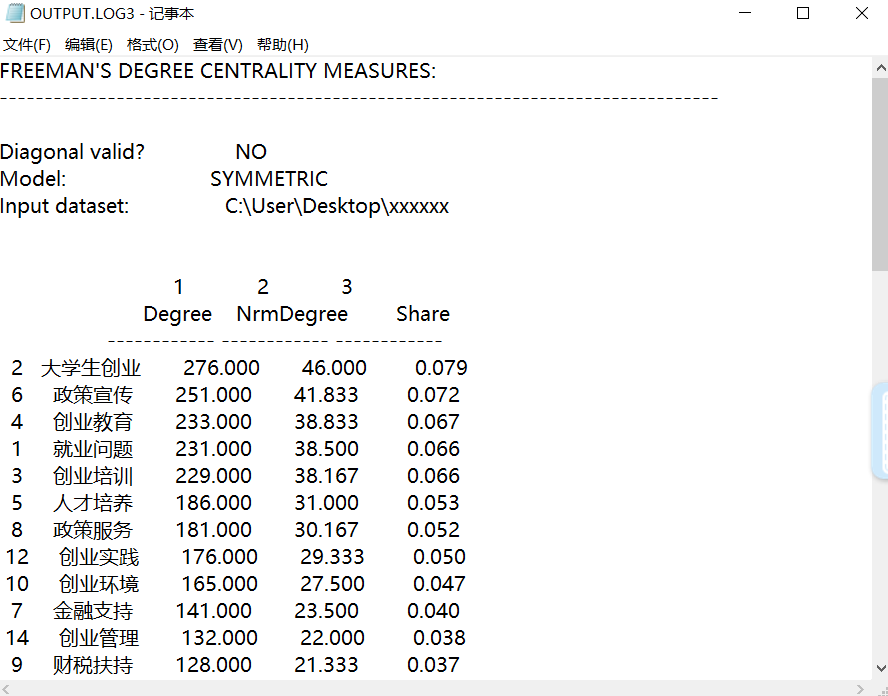closeness centrality：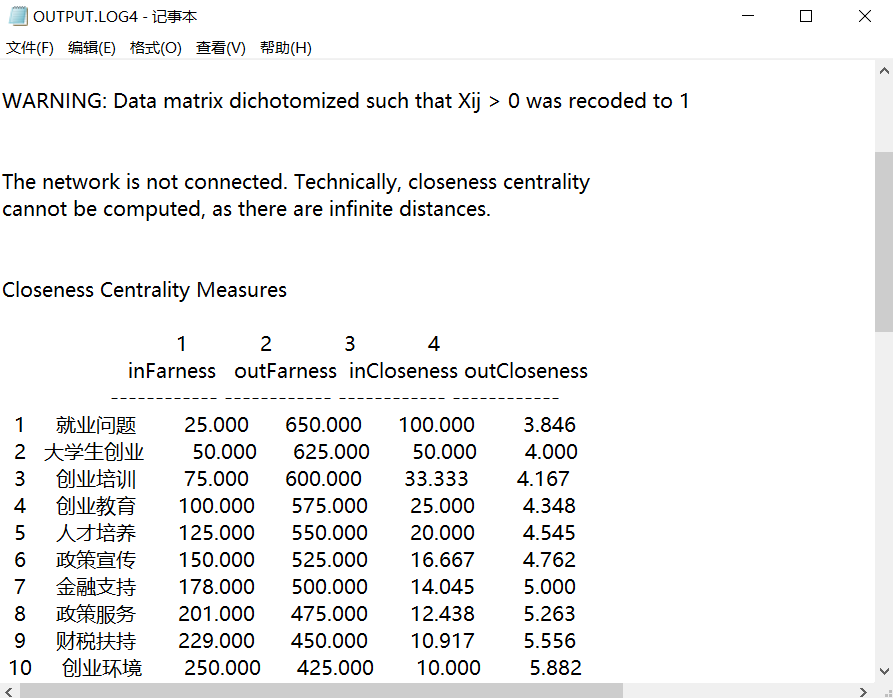然后关于betweenness centrality，一般都是选择Node betweenness ，其它两种lines和Hierarchically Reduction 我也没有在实际建模中用过，不太清楚有啥具体意义，而且这两种没有显示完各个点中心性值后的探索性分析的表格，关于一些平均值、方差、标准差等，所以这里直接拉到第一个就行了。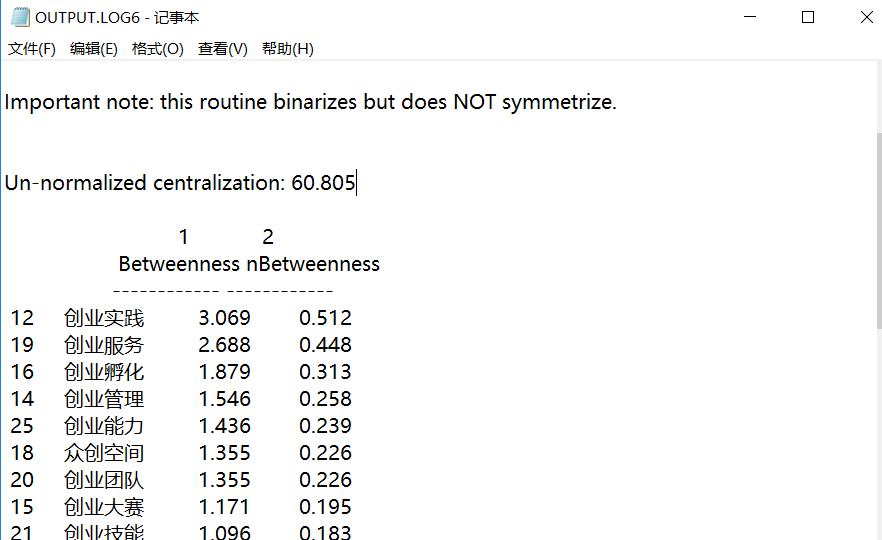关于上述三张表所能给出的信息，我们都可以通过分析他们按高位降序排序的指标来进一步得出结果，其中还是以Degree centrality为例，因为我没有进行二值化，或者说排除一些其它类型的指标，另外就是当时晚上时间匆忙，现在第二天是接着昨晚的写，一般如果数据做了处理，当我们做了这个中心性后，Num值的区间都是在0 ~ 100之间，排在最前的Num一定是在95%左右再降序，这样才能根据降序来定义最后的指标区别，这里倒是有些无法判断。其余两个中心性的解释根据公式和网络分析图结合起来说明更加有真实性与连贯性，在建模中，这就是基本的网络分析了。

4、凝聚子群分析
凝聚子群是满足如下条件的-一个行动者子集合，即在此集合中的行动者之间具有相对较强、直接、紧密、经常的或者积极的关系。城市网络凝聚子群是用于揭示和刻画城市群体内部子结构状态。找到城市网络中凝聚子群的个数以及每个凝聚子群包含哪些城市成员，分析凝聚子群间关系及联接方式，这都可以从新的维度考察城市群网络的发展状况。
利用ucinet软件中的CONCOR法进行凝聚子群分析。CONCOR是-种迭代相关收敛法( convergent correlation或者convergence of iterated correlation)。它基于如下事实:如果对-一个矩阵中的各个行(或者列)之间的相关系数进行重复计算(当该矩阵包含此前计算的相关系数的时候)，最终产生的将是一个仅由1和-1组成的相关系数矩阵。进一步说我们可以据此把将要计算的一些项目分为两类:相关系数分别为1和-1的两类(刘军，2009)。
这个我不太记得具体有啥作用了，找自己之前写过的网络分析法中很少用凝聚子群，但确实用过所以我现在才有一个大致的印象，然而怎么解释已经忘了，都过了一年多了，所以尴尬了，还是在这里记录一下吧，下次记起来回来补。
凝聚子群分析结果：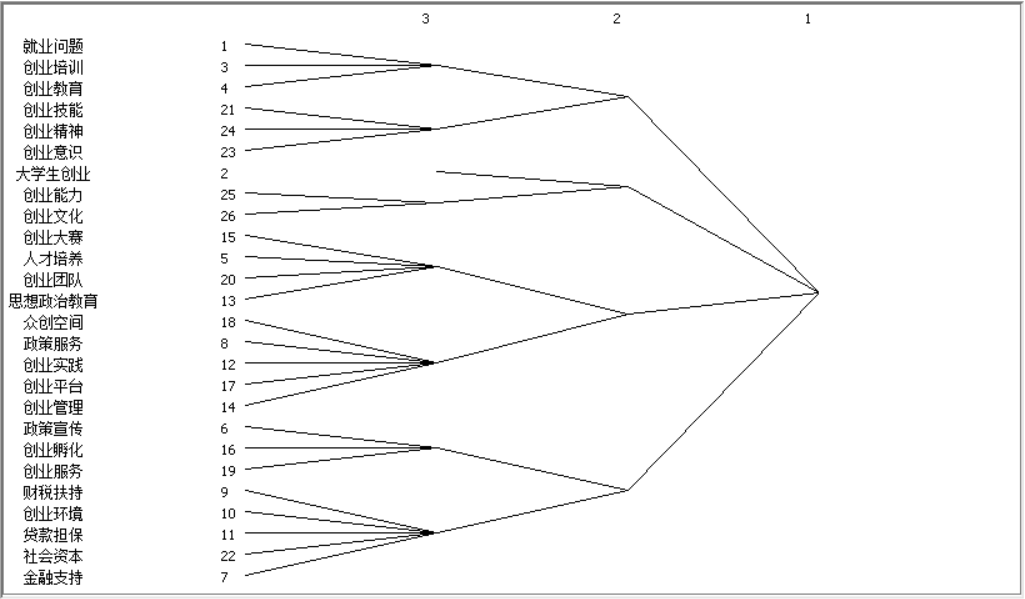凝聚子群分析结果：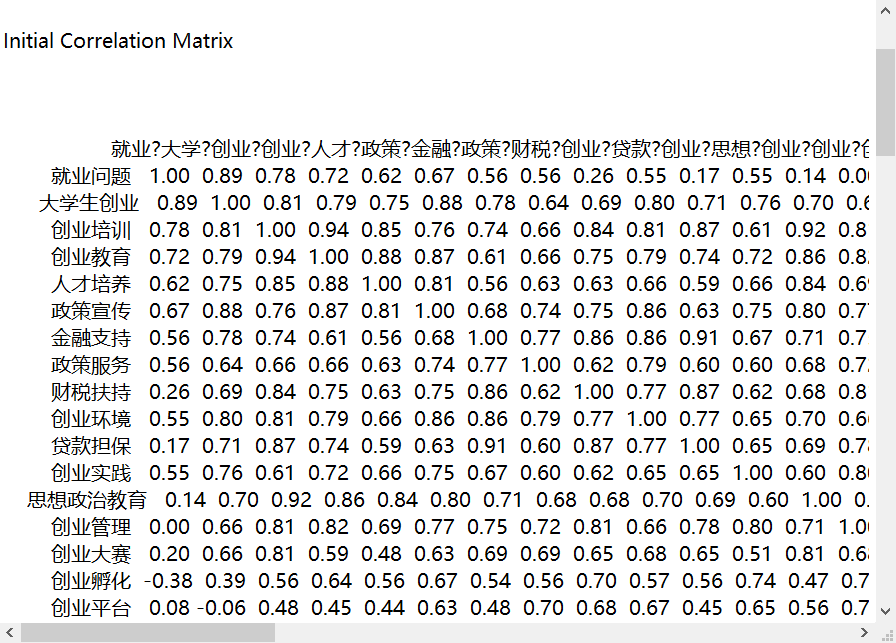总结
其实抛开一些太专业性的东西不讲，本篇博文写得挺爽的，很久没有敲过这么多文字以及再次以一种建模的心理完成对ucinet的一些基本操作介绍，起因是一个建模群的网友问我网络分析里的一些技巧，还有他用python做的话好不好做，于是我去搜了一下，找到了本篇的第一篇参考文献，看了下python确实可以做但比较麻烦，我没用过，而ucinet的话，脑子一闪，发现这不就是我大学一直在用的东西嘛，伴随着的还有spss statistics、spss modeler、MATLAB、PS、灰色系统软件、yaahp等等各种零零碎碎的东西，突然就有些怀旧，虽然刚出来一年多，由大学学的电气跨进计算机的道路，终是专业化了，但想想曾经大二一年里在建模路上的摸爬滚打，每次和队友完成一篇篇论文到获奖的喜悦，也终是我如今很难获取到的了。所以，且行且珍惜吧，end。

参考与推荐： . 六个主要的社会网络分析软件的比较
. 网络的介数中心性(betweenness)及计算方法
. SNA(社会网络分析)——三种中心度总结
. 《社会网络分析法》(刘军 译)
. 薛萍. 基于社会网络的研究型高校优势学科方向发展预测研究 [D]. 导师: 朱凌. 浙江大学,2014
. 社会网络分析方法简介
展开全文• 前言： 目前，许多的web应用，或者web接口，都会在服务器的入口处，使用一个服务器容器来监听端口，...今天要讨论的重点为在服务器集群中，合理使用nginx的hash策略做更有意义的负载均衡。 概要： 当我们的服务
前言：

目前，许多的web应用，或者web接口，都会在服务器的入口处，使用一个服务器容器来监听端口，然后进行请求转发，例如nginx Apache等。

服务器容器对应整个web服务有着至关重要的作用，包括：可以很好的管理服务进程，进行代理，对请求的预处理，以及负载均衡。

今天要讨论的重点为在服务器集群中，合理使用nginx的hash策略做更有意义的负载均衡。

概要：

当我们的服务是由一台服务器支撑时，就丝毫不存在负载均衡的概念。只有当服务由多台服务器（也就是服务器集群）支撑时，才会使用到负载均衡。

负载均衡顾名思义，是一种策略，用于防止一台服务器过载，而其他服务器闲置情况发生的策略。通过该策略可以使得提供相同服务的服务器负载基本相同。说得直白一点，就是当客户端发起一个请求之后，负载均衡会通过预先设定好的策略将该请求转发给上游的一台服务器进行处理。

如图所示：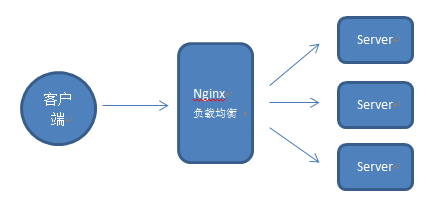负载均衡是一个很成熟的技术，其中对后端服务器进行轮询；通过客户端请求IP进行hash；对后端服务器指定权重等，是较为常见的负载均衡策略。这里不再赘述。

对服务盲目的采用负载均衡策略，是不太合理的。负载均衡默认情况下是轮询策略，这在一些场景下并不高效。

更有意义的负载均衡：

今天讲解的重点是，两种常见的负载均衡hash策略，以及对应的使用场景。

1、ip_hash（通过客户端请求ip进行hash，再通过hash值选择后端server）:

当你服务端的一个特定url路径会被同一个用户连续访问时，如果负载均衡策略还是轮询的话，那该用户的多次访问会被打到各台服务器上，这显然并不高效（会建立多次http链接等问题）。甚至考虑一种极端情况，用户需要分片上传文件到服务器下，然后再由服务器将分片合并，这时如果用户的请求到达了不同的服务器，那么分片将存储于不同的服务器目录中，导致无法将分片合并。所以，此类场景可以考虑采用nginx提供的ip_hash策略。既能满足每个用户请求到同一台服务器，又能满足不同用户之间负载均衡。

配置代码如下：
upstream backend{
ip_hash;
server 192.168.128.1:8080 ;
server 192.168.128.2:8080 ;
server 192.168.128.3:8080 down;
server 192.168.128.4:8080 down;
}
server {
listen 8081;
server_name test.csdn.net;

root /home/system/test.csdn.net/test;

proxy_pass http://backend;
}

}上述是一个极简的监听8081端口的的nginx服务，其中当请求url 为/Upload/upload时，会走ip_hash策略; upstream是nginx的负载均衡模块，此处，配置了策略为ip_hash，参与负载均衡的机器有四台，其中后两台末尾添加了down关键字，表示下线的意思。

2、url_hash（通过请求url进行hash，再通过hash值选择后端server）：

一般来讲，要用到urlhash，是要配合缓存命中来使用。举一个我遇到的实例：有一个服务器集群A，需要对外提供文件下载，由于文件上传量巨大，没法存储到服务器磁盘中，所以用到了第三方云存储来做文件存储。服务器集群A收到客户端请求之后，需要从云存储中下载文件然后返回，为了省去不必要的网络带宽和下载耗时，在服务器集群A上做了一层临时缓存（缓存一个月）。由于是服务器集群，所以同一个资源多次请求，可能会到达不同的服务器上，导致不必要的多次下载，缓存命中率不高，以及一些资源时间的浪费。在此类场景下，为了使得缓存命中率提高，很适合使用url_hash策略，同一个url（也就是同一个资源请求）会到达同一台机器，一旦缓存住了资源，再此收到请求，就可以从缓存中读取，既减少了带宽，也减少的下载时间。

配置代码如下：

upstream somestream {
hash $request_uri; server 192.168.244.1:8080; server 192.168.244.2:8080; server 192.168.244.3:8080; server 192.168.244.4:8080; } server { listen 8081 default; server_name test.csdn.net; charset utf-8; location /get { proxy_pass http://somestream; } }上述同样也是一个极简的监听8081端口的nginx服务，当请求url为/get时，会走url_hash；同样配置了upstream模块，hash$request_uri表明了是按照url规则进行hash策略。

总结：

以上就是本文要介绍的全部内容，本文侧重讲解了ip_hash和url_hash的使用场景和基本配置，另外，在进行nginx server 配置时，可以灵活一些，不同的location采用不同的策略，可以使得服务策略更加的合理。希望此文能为各位带来些许帮助。


展开全文nginx 负载均衡 web服务
• 卷积神经网络原理 ...学习本课程之前，请先学习课程814 使用python实现深度神经网络以了解必要的基本概念，本实验中涉及到的深度学习基本概念将不会再次阐述。同时建议学习课程744 深度学习初探——入门

卷积神经网络原理
一、实验介绍
1.1 实验内容
本课程将会先带你理解卷积神经网络的原理，了解卷积神经网络的一些特性。然后动手使用caffe深度学习框架训练一个卷积神经网络模型，并用训练好的模型进行图片分类。
学习本课程之前，请先学习课程814 使用python实现深度神经网络以了解必要的基本概念，本实验中涉及到的深度学习基本概念将不会再次阐述。同时建议学习课程744 深度学习初探——入门DL主流框架(该课为训练营课程)中的caffe相关部分，但本课程中涉及到caffe的内容也会尽量讲解。
本实验作为本课程的第一次实验，将会向大家讲解卷积神经网络的相关基本原理。除非你已经知道什么是卷积神经网络，否则我强烈建议你仔细学习本次实验，理解原理将非常有助于你在实际问题中合理地使用卷积神经网络。
1.2 实验知识点
卷积神经网络的结构
1.3 先学课程
814 使用python实现深度神经网络(必学，否则可能无法理解本课程中的一些概念)744 深度学习初探——入门DL主流框架(选学)
1.4 实验环境
python 2.7caffe 1.0.0 (实验楼环境中已经预先安装)
二、实验步骤
2.1 什么是“卷积”
在课程814 使用python实现深度神经网络中，我们已经构建过深度神经网络，注意在那个网络中，除了激活函数层和损失函数层之外，发挥主要作用的是全连接层，我们的网络参数全部都在全连接层中。那么既然使用全连接层构成的神经网络就已经实现了对图片的分类，为什么我们还需要“卷积神经网络”呢？为了回答这个问题，我们先来了解到底什么是卷积(convolution)。
2.1.1 卷积操作
“卷积”这一词在多个领域里都有定义（比如信号处理领域的傅里叶变换中也有卷积）。具体在图像处理领域，卷积操作是指使用一个小的“模板窗口”对一个图片中的所有与模板大小相同的区域进行“卷积运算”。“卷积运算”其实很简单，就是将模板中的每一个数字与图片中相同大小区域的对应数字（像素值）进行相乘，再求和。具体操作如下图：“模板窗口”每移动到一个位置，就和图片中的对应元素进行一次卷积运算，注意我们一般把“模板窗口”称为卷积核(kernel)。
比如在第一个位置，图片上的四个像素值为[[0,0],[1,1]], 而卷积核中的数值为[[-1,1],[-1,1]], 对应元素相乘再求和，得到0*-1+0*1+1*-1+1*1=0 比如在第二个位置，图片上的四个像素值为[[0,0],[1,0]], 对应元素相乘再求和，得到0*-1+0*1+1*-1+0*1=-1
对图片中的所有可能位置进行卷积操作，就得到了最终的卷积结果。
2.1.2 为什么卷积操作适合用来处理图像
上图中的卷积核，其实是经过精心设置的。观察被卷积的图像和卷积结果你会发现，该卷积核其实可以用来检测图片中的垂直边缘。如果卷积后得到的数字绝对值大，就说明图片上的对应地方有一条垂直方向的边缘（即像素数值变化较大）。如果卷积后得到的数字绝对值很小，则说明图片上的对应地方像素值变化不大，没有边缘存在。同样的道理，你可以构造一个检测水平方向边缘的卷积核:你可以尝试用这个卷积核去对上面的图片进行卷积操作，验证这个卷积核是不是检测出了水平方向的图片边缘。 卷积操作还可以有更多强大的功能，在数字图像处理中经常会用到。
说到这里，你也许已经能够体会为什么我们需要卷积神经网络。对于将整个图片视为一个向量的全连接层来说，它忽略了图像本身所具有的的“二维空间特性”，或者说局部特性。而卷积操作则十分擅长处理这种局部特性，能够更有效地提取出图片中的更多有用信息（比如图片中的边缘）。实际上，卷积神经网络几乎已经成为了神经网络中处理图像的标配。
2.2 神经网络中的卷积层
在具体的将卷积操作运用到神经网络的过程当中，有许多细节需要注意（包括很多超参数）。首先需要明确的是，和课程814 使用python实现深度神经网络中介绍的一样，卷积层中的参数（即卷积核中的每个数字）也是通过学习算法（随机梯度下降算法）学习得到的，不需要手工设定。
2.2.1 特征个数
一个卷积核，只能检测一种特征(feature)（比如垂直方向的边缘），而图片中的信息往往很复杂，一个卷积核显然不够。所以神经网络中，一个卷积层往往会有多个卷积核，这样卷积层的输出就会有多层。如下图：为了简便，在一些深度学习框架（比如本课程会用到的caffe）中，在运算时可以将特征个数与图像通道数等同看待。所以对于一个通道数为1的图片，若卷积层特征个数为3，则可将输出的卷积结果视为通道数为3的图片。对于一个通道数为3的图片（此时，卷积核是一个三维的“体”），若卷积层特征个数为16，则可将输出的卷积结果视为通道数为16的图片。
2.2.2 stride
上面的示意图中，卷积核在水平和垂直方向上每次都移动一个像素的距离，在实际的卷积神经网络中，卷积核可能一次移动不止一个像素。卷积核每次移动的“步长”被称为stride。stride的大小会影响最后卷积结果的大小。比如将水平和垂直方向的stride都从1改成2，原来的示意图就会变成这样：2.2.3 卷积核尺寸(kernel size)
上面的示意图中卷积核的尺寸是2x2, 但在实际当中，很少见到边长为偶数的卷积核。且一般卷积核的最小边长为3（也存在边长为1的卷积核，你也许会觉得奇怪，边长为1的卷积层与全连接层岂不是几乎没有区别了，这个问题保留在这里，我们以后也许会来回答这个问题）。
观察上面的图片你会发现，卷积操作得到的卷积结果与原来的图片相比尺寸变小了。这样会带来一个问题，有的卷积神经网络会非常“深”（比如几十层，甚至上百上千层）如果每经过一个卷积层我们的图片都变小一点，那到最后，有可能我们的图片都没有卷积核大，没办法继续卷积下去了。为了解决这个问题，人们对卷积层引入了边缘填充(padding)：在卷积前，先将图片扩大一点点，然后再进行卷积。对于扩大部分，一般会填入0值。如下图（为了作图方便，卷积核与卷积结果之间只使用了一个箭头进行指示）：2.2.5 计算卷积结果的尺寸
对于一个尺寸为w*w的正方形图片，卷积结果的尺寸（假设其尺寸是o*o），会受到上面的特征个数feature、步长stride、卷积核尺寸kernel、边缘padding四个超参数的影响。你可以尝试一下推导卷积结果尺寸的公式，其实很简单：
o=(w+2*padding-kernel)/stride+1 且输出结果的通道数等于feature
这个公式只适合于输入图片为正方形，卷积核也为正方形，步长在水平和垂直方向一致，padding在四个方向都一样的情况（不满足这些条件的情况你可以自己推导）。比如对于尺寸为17*17的输入图片，padding为1，kernel的尺寸为3*3, stride为1，则卷积之后的输出边长为：(17+2*1-3)/1+1=17，没有变化。
注意根据上面的公式，你会发现卷积层的几个超参数之间需要满足如下关系: (w+2*padding-kernel)%stride==0 其中的%为取模符号，即(w+2*padding-kernel)需要是stride的整数倍，不然无法除尽。
2.3 卷积层的实现
卷积层相对全连接层来说比较复杂（从其中超参数的个数很多你就能看出来）。你可以接着课程814尝试自己使用python实现卷积层，其实原理都是一样的，关键都是运用链式法则去求梯度。不过除非你有特别的兴趣，不然不推荐自己“造轮子”（作者自己造了一个，如果你对代码有兴趣，请到Github查看）。幸运的是好多现有的深度学习框架已经帮我们实现好了卷积层（比如本课程会用到的caffe），直接拿过来用就好了。
三、实验总结
本次实验我们学习了卷积操作的基本概念。掌握了这些基本概念，之后的实验对你来说会非常容易。如果你对其中的一些概念还感到困惑，不用感到受挫，刚开始接触新东西都会有这种感觉。请你再仔细思考一遍这些概念的含义，然后去继续做之后的实验，当碰到相应的概念时就回过头来看一看，相信这些对你来说都不难掌握。
本次实验，我们学习了：
四、课后作业
以下的作业都能根据上面的文档推测出答案。

展开全文图像分类
• 译者在自动化部署的时候遇到了很多...本文为博主翻译文章，转载请注明出处Bob Hou: http://blog.csdn.net/minxihou 本指南将会引导你如何配置openstack网络节点，网络节点会使用到ML2插件和OpenvSwitch机制。1 单网卡的openstack 插件 devstack
• 1) 安装并使用VMware虚拟机。 2) 练习Win系统下的常见网络命令（ping、net、at...要求学生使用扫描器软件进行扫描，对扫描的结果作出正确的分析，根据分析评估网络系统安全性，必要时关闭危险端口，合理配置计算机系统
• 本代码使用监督学习的方法来更合理的设置参数取值，设置神经网络参数的过程就是神经网络的训练过程。使用监督学习的方式设置神经网络参数需要有一个标注好的训练数据集batch，监督学习最重要的思想就是，在已知答案...前向传播 反向传播 深度学习 python
• 使用caffe构建卷积神经网络 一、实验介绍 1.1 实验内容 上一次实验我们介绍了卷积神经网络的基本原理，本次实验我们将学习如何使用深度学习框架caffe构建卷积神经网络，你将看到在深度学习框架上搭建和训练模型是...caffe
• 有很多人使用hettp请求网络的时候，总是想问，get和post那个好啊？他们的区别是什么？甚至一些面试题也是这样写的！所以我搜了一些资料，众说纷纭啊，以至于我自己都不确定自己找的资料是否靠谱1、get和post区别，...Http get post
• 在我们之前的网络测试中，主要是限制网速来模拟弱网络环境，而实际中弱网络时，网络延时，丢包率都会有变化，所以使用网速+丢包率+延时来定义一种网络环境更加合理 本篇文章介绍ATC工具环境的搭建和使用，搭建方法...
• clumsy 能在 Windows 平台下人工造成不稳定的网络状况，方便你调试应用程序在极端网络状况下的表现。 简介 利用封装 Winodws Filtering Platform 的WinDivert 库, clumsy 能实时的将系统接收和发出的网络数据包...
• (1) 熟悉 ethereal 的使用 (2) 验证各种协议数据包格式 (3) 学会捕捉并分析各种数据包。 二、使用仪器、器材 Wireshark软件，window10系统计算机 三、实验内容及原理 （1） 安装 ethereal 软件 （2） 捕捉数据包，...c++ c#
• 在所有威胁情报子类型中，开源网络情报（OSINT）也许是使用最广泛的情报，这是有道理的。毕竟，它大部分都是免费的，谁能拒绝呢？ 不幸的是，就像其他主要的子类型（人类情报，信号情报和地理空间情报，仅举几例）...搜索引擎
• 交通预见未来(6) 使用卷积神经网络做交通速度预测 1、文章信息 《Learning Traffic as Images: A Deep Convolutional Neural Network for Large-Scale Transportation Network Speed Prediction》。 北航马晓磊...交通速度预测
• pacman -S iproute2 网络管理的包还是要安装的不然太麻烦了 systemctl enable dhcpcd 设置一下有效方便下次就不用再启动了 后查网络问题的时候 发现用systemd-networkd就够了不需要什么dhcpcd dhcp网络的话...
• Python中使用LSTM网络进行时间序列预测 2017-04-09 21:08来源:AI100 原标题：AI 技术讲座精选：Python中使用LSTM网络进行时间序列预测 长短记忆型递归神经网络拥有学习长观察值序列的潜力。 它似乎是实现时间...python
•  高度地封装, 提供一些简单易用的 API 方便我们在 iOS 开发中发出网络请求并在其上更快地构建网络层组件并提供合理的接口. 到这里，这一篇文章从上到下对 AFNetworking 是如何调用的进行了一个简单的概述，我...ios开发 ios
• 深度学习并没有你想象的那么难，本课程将会一边讲解深度学习中的基本理论，一边通过动手使用python实现一个简单的深度神经网络去验证这些理论，让你从原理上真正入门深度学习。 本次实验将会带大...
• workgroup无法访问 您可能没有权限使用网络资源 （解决方案）
• 内部网络全部使用10.186.x.x网段，SW2和SW3两个交换机连接两个学院， Sw2学院有电脑500台，sw3学院有电脑200台，合理分配IP地址段，避免浪费， 两个学院可以互相访问，也可以访问内网的web server， 分别对两个学院...
• 三层网络设计的结构发展已经很成熟，但leaf-spine (leaf叶节点，spine脊节点)结构越来越热门，网络设计师们应该如何进行选择呢? 尽管三层网络结构应用广泛而且技术成熟，但随着技术的发展，它的瓶颈也不断涌现，...
• 防火墙是近几年发展起来的一种保护计算机网络安全的技术措施,也是目前使用最广泛的一种网络安全防护技术。本文重点从防火墙的配置在网络安全中的重要性方向出发,阐述了防火墙规则集是防火墙产品安全的重要措施。...